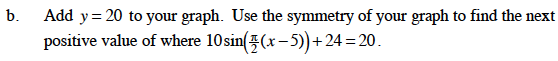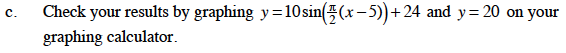### Home > PC > Chapter 6 > Lesson 6.2.2 > Problem6-86

6-86.
1. Now consider the equation 10sin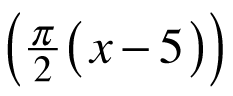+ 24 = 20. Homework Help ✎

1. Solve the equation for the smallest positive value of x (in radians). If you are having a problem getting started, let u =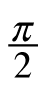(x − 5). Be sure to choose the correct value of x.

2. Add y = 20 to your graph. Use the symmetry of your graph to find the next positive value of where 10sin+ 24 = 20.

3. Check your results by graphing y = 10sin+ 24 and y = 20 on your graphing calculator.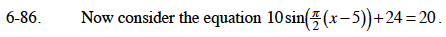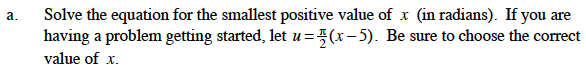Substituting 'u' into the equation.
10sin(u) + 24 = 20
10sin(u) = −4

Using a calculator, u = −0.4115.
Substitute back to solve for x.